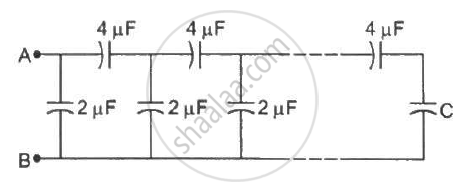Department of Pre-University Education, KarnatakaPUC Karnataka Science Class 12
Share

# A Finite Ladder is Constructed by Connecting Several Sections of 2 µF, 4 µF Capacitor Combinations as Shown in the Figure. - Physics

ConceptCapacitors and Capacitance

#### Question

A finite ladder is constructed by connecting several sections of 2 µF, 4 µF capacitor combinations as shown in the figure. It is terminated by a capacitor of capacitance C. What value should be chosen for C, such that the equivalent capacitance of the ladder between the points A and B becomes independent of the number of sections in between?#### Solution

The equivalent capacitance of the ladder between points A and B becomes independent of the number of sections in between when the capacitance between A and B is C.

The capacitors C and 4 µF are in series; their equivalent capacitance is given by C_1 = (C xx 4) / (C+4)

The capacitors C1 and 2 µF are in parallel; their equivalent capacitance is given by C = C1 + 2 µF

⇒ C = (C xx 4) / (C+4) + 2

⇒ 4C + 8 + 2 C = 4C + C2

⇒ C2 − 2C − 8 = 0

⇒ C = −2, C = 4

Capacitance cannot be negative.

∴ C = 4 µF

The value of C is 4 µF.

Is there an error in this question or solution?

#### Video TutorialsVIEW ALL 

Solution A Finite Ladder is Constructed by Connecting Several Sections of 2 µF, 4 µF Capacitor Combinations as Shown in the Figure. Concept: Capacitors and Capacitance.
S